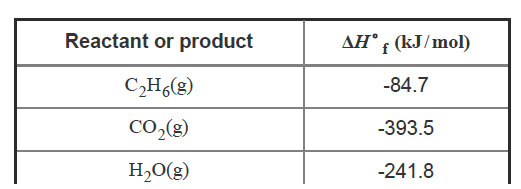# Problem: Consider the exothermic reaction2 C2H6(g) + 7 O2(g) → 4 CO2(g) + 6 H2O(g)Calculate the standard heat of reaction, or ΔH°rxn, for this reaction using the given data. Also consider that the standard enthalpy of the formation of elements in their pure form is considered to be zero.Express your answer to three significant figures and include the appropriate units.Express your answer to four significant figures and include the appropriate units.Calculation of the heat of reaction based on the standard heat of formation The enthalpy change for the formation of 1 mol of a substance in its standard state from its constituent elements in their standard states is called the standard heat of formation. It is denoted as ΔH°f. If the standard heat of formation of the reactants and the products is known, the standard heat of reaction can be calculated using the formula ΔH°rxn = ∑npΔH°f(products) − ∑ nrΔH°f(reactants)Here, np is the total number of moles of products, and nr is the total number of moles of reactants.

###### FREE Expert Solution
99% (80 ratings)View Complete Written Solution
###### Problem Details

Consider the exothermic reaction

2 C2H6(g) + 7 O2(g) → 4 CO2(g) + 6 H2O(g)

Calculate the standard heat of reaction, or ΔH°rxn, for this reaction using the given data. Also consider that the standard enthalpy of the formation of elements in their pure form is considered to be zero.

Express your answer to three significant figures and include the appropriate units.Express your answer to four significant figures and include the appropriate units.

Calculation of the heat of reaction based on the standard heat of formation

The enthalpy change for the formation of 1 mol of a substance in its standard state from its constituent elements in their standard states is called the standard heat of formation. It is denoted as ΔH°f.

If the standard heat of formation of the reactants and the products is known, the standard heat of reaction can be calculated using the formula

ΔH°rxn = ∑npΔH°f(products) − ∑ nrΔH°f(reactants)

Here, np is the total number of moles of products, and nr is the total number of moles of reactants.